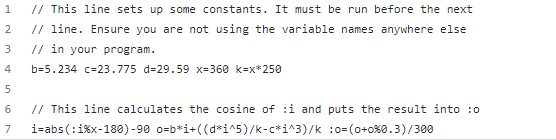# cosine help

##### Veteran endo
i found this github site that had a yolol code for figuring the cosine value with an advanced chip, as professional chips are not really in game yet...
github.com/CylonSB/Scripts/tree/master/Scripts/Cosine%20(Basic%20Chip)

// This line sets up some constants. It must be run before the next
// line. Ensure you are not using the variable names anywhere else
b=5.234 c=23.775 d=29.59 x=360 k=x*250
// This line calculates the cosine of :i and puts the result intoi=absi%x-180)-90 o=b*i+((d*i^5)/k-c*i^3)/k=(o+o%0.3)/300so I'm trying the get this to work, but it is not.
the problem is the (o+o%0.3) part
the wiki is horrible at anything else other than basic info
I don't know what "%" is in yolol and I'm having trouble figuring it out
if i had more informed wiki i mite be able to figure out a workaround to this problem but this is not the case.
anyone know how to make this work, or is it a bug in yolol chips for "%" not working with a decimal numbers?

Last edited:

#### Womble

##### Veteran endo
I would guess* that the "%" is a typo. It's looks like it's meant to be an operator; I'd try substituting in ^, *, /, + and - one at a time in that order (based on my perceived likelihood that those characters are typoes of %, basically; it's trial-and-error, so doesn't really matter) to see if any of them make it work.

If none of them do, you might get some joy out of Googling for "how to calculate COSINE without tables or a calculator", and trying to puzzle out what that part of the line is doing. If you want to stretch your math muscles...* I am neither a mathematician nor an expert on YOLOL...

##### Veteran endo
I would guess* that the "%" is a typo. It's looks like it's meant to be an operator; I'd try substituting in ^, *, /, + and - one at a time in that order (based on my perceived likelihood that those characters are typoes of %, basically; it's trial-and-error, so doesn't really matter) to see if any of them make it work.

If none of them do, you might get some joy out of Googling for "how to calculate COSINE without tables or a calculator", and trying to puzzle out what that part of the line is doing. If you want to stretch your math muscles...* I am neither a mathematician nor an expert on YOLOL...
the first "%" in the code works, it dose something. if i change the %0.3 to %3 the code works, though i expect that the math is way wrong when i do this.
i just dont know that "%" is doing....

#### DankHoneyOil

##### Active endo
the first "%" in the code works, it dose something. if i change the %0.3 to %3 the code works, though i expect that the math is way wrong when i do this.
i just dont know that "%" is doing....
% is the modulo function. Google can probably help you figure out what that means better then I can explain it.

#### Greebo

##### Learned-to-turn-off-magboots endo
% is a real operator like dank said.

In this case it's saying divide o by 0.3, but give me decimal remainder.

An example is 1%0.3 = 0.333

1 / 0.3 is 3.333. in the case of %, it would be .333, or just the reminder

#### Agonarch

##### Active endo
I came across an approximation that's not too bad, if you still haven't gotten this to work, try;
Code:
``i=:x/180 :sinx=4*i*(1-ABS i) i+=.5-2*(i>.5) :cosx=4*i*(1-ABS i)``

##### Veteran endo
I came across an approximation that's not too bad, if you still haven't gotten this to work, try;
Code:
``i=:x/180 :sinx=4*i*(1-ABS i) i+=.5-2*(i>.5) :cosx=4*i*(1-ABS i)``
i can make it work fine with Professional chips, but to do a sinx or cosx you need a Professional chip... they can not be made in game yet, and buying 12 of them is not going to happen..
i did finally come across this